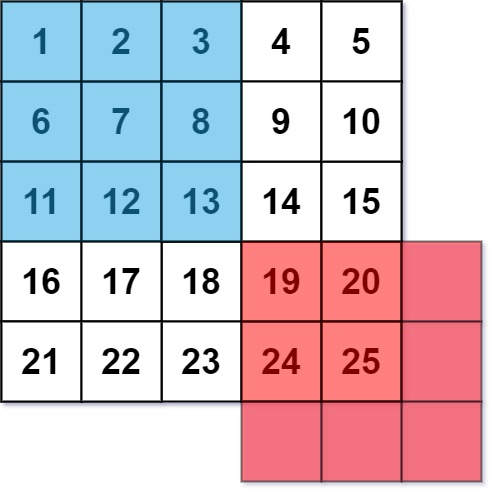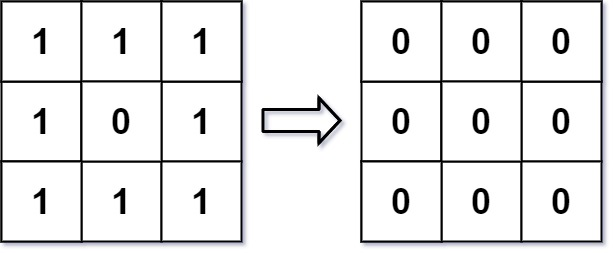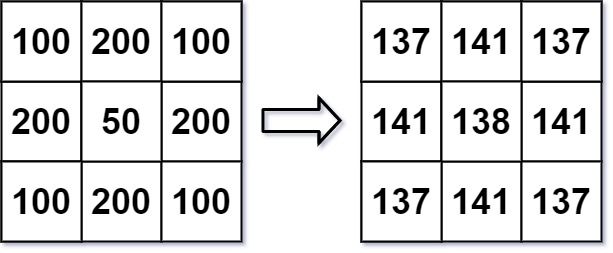# GeetCode Hub

An image smoother is a filter of the size `3 x 3` that can be applied to each cell of an image by rounding down the average of the cell and the eight surrounding cells (i.e., the average of the nine cells in the blue smoother). If one or more of the surrounding cells of a cell is not present, we do not consider it in the average (i.e., the average of the four cells in the red smoother).Given an `m x n` integer matrix `img` representing the grayscale of an image, return the image after applying the smoother on each cell of it.

Example 1:```Input: img = [[1,1,1],[1,0,1],[1,1,1]]
Output: [[0,0,0],[0,0,0],[0,0,0]]
Explanation:
For the points (0,0), (0,2), (2,0), (2,2): floor(3/4) = floor(0.75) = 0
For the points (0,1), (1,0), (1,2), (2,1): floor(5/6) = floor(0.83333333) = 0
For the point (1,1): floor(8/9) = floor(0.88888889) = 0
```

Example 2:```Input: img = [[100,200,100],[200,50,200],[100,200,100]]
Output: [[137,141,137],[141,138,141],[137,141,137]]
Explanation:
For the points (0,0), (0,2), (2,0), (2,2): floor((100+200+200+50)/4) = floor(137.5) = 137
For the points (0,1), (1,0), (1,2), (2,1): floor((200+200+50+200+100+100)/6) = floor(141.666667) = 141
For the point (1,1): floor((50+200+200+200+200+100+100+100+100)/9) = floor(138.888889) = 138
```

Constraints:

• `m == img.length`
• `n == img[i].length`
• `1 <= m, n <= 200`
• `0 <= img[i][j] <= 255`

class Solution { public: vector<vector<int>> imageSmoother(vector<vector<int>>& img) { } };